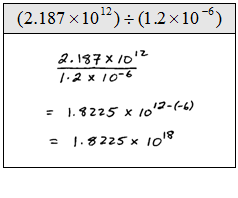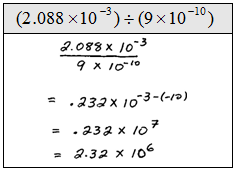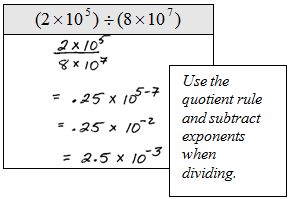## Pages

### Negative Exponents

The quotient rule for exponents can be used to define negative exponents. It might seem strange to think of negative exponents, but we need to know where they come from and how to work with them.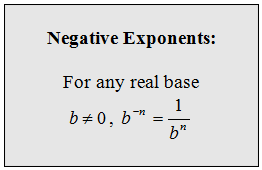Factors in the numerator with a negative exponents move to the denominator.Simplify.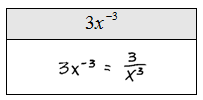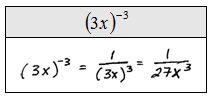If you are given a factor with a negative exponent in the denominator simply move it to the numerator. Use the following reasoning to justify this.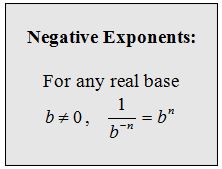Simplify.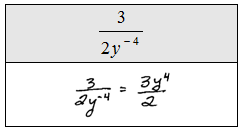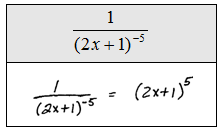A common mistake is to multiply the base with the exponent when it is negative. For example,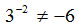Avoid this mistake . The correct solution isAnother useful property involves a rational expression raised to a negative exponent.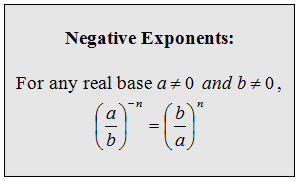Simplify.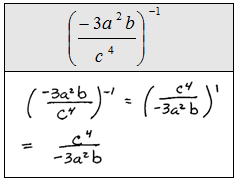When simplifying expressions, it usually is best to simplify within the parentheses first and then apply the product and/or the quotient rule.

Simplify.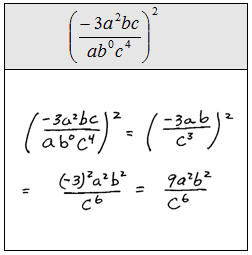Scientific notation is an application of negative exponents. It is used to express very large or very small numbers.An example of a power of ten might look like,Use this to convert number expressed in scientific notation to a decimal.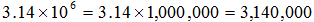Remember that we can obtain this same result by moving the decimal over six places to the right and filling in with the digit 0.

A power of ten might be negative,Negative exponents appear when working with very small numbers.Obtain the same result by moving the decimal over to the left 4 places.

Express the number in scientific notation.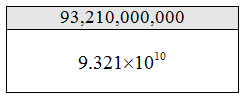Choose to place the decimal so that the first digit is between 1 and 10.

Multiplication is commutative, so when multiplying numbers in scientific notation multiply the decimal parts first. Next, multiply the powers of 10 using the product rule.

Multiply.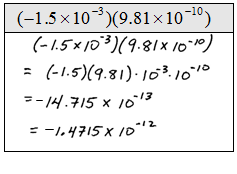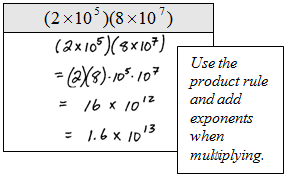Divide.